• 两个重要的排序算法的时间复杂度比较。所用的代码比较简陋，使用控制台。
• 时间复杂度: 用T(n)T(n)T(n)表示排大小为nnn的数组的时间:T(n)=2T(n/2)+nT(1)=1T(n)=2T(n/2)+n T(1)=1T(n)=2T(n/2)+nT(1)=1 关于如何解这个递归方程，这里介绍其中一种，我们假设n是2的幂: 首先，我们递推关系式的...
归并排序

归并排序：利用分治的思想，先排左边一半，再排右边一半，最后再将两边有序的合并起来。
时间复杂度: 用$T(n)$表示排大小为$n$的数组的时间：$T(n)=2T(n/2)+n\quad T(1)=1$

关于如何解这个递归方程，这里介绍其中一种，我们假设n是2的幂:

首先，我们递推关系式的两边同时除以n，得到:$\frac{T(n)} {n}=\frac{T(n/2)}{n/2}+1$。
因为该方程对2的幂的任意n是成立的，于是还可以写成：
$\frac{T(n/2)}{n/2}=\frac{T(n/4)}{n/4}+1$
$\frac{T(n/4)}{n/4}=\frac{T(n/8)}{n/8}+1$
…
$\frac{T(2)}{2}=\frac{T(1)}{1}+1$
此时，我们把这些式子的左边和右边统一加起来，会发现很多项都同时消掉了，我们称之为叠缩公式。
最后剩下:$\frac{T(n)}{n}=\frac{T(1)}{1}+logn$，此时$T(n)=nlogn+n=O(nlogn)$

快速排序

快速排序的主要思想依然是分治：
1、从待排数组中选出一个枢纽元（$pivot$）；
2、然后将数组分割为两个不相交集合$s1,s2$，左半边集合的元素都小于等于$pivot$，右半边集合元素都大于等于$pivot$。
3、最后，分别排序左半边、右半边。

快速排序的枢纽元选择策略一般为：
1、三数中值分割法：即选择待排数组的左段、右段和中心位置的中值作为枢纽元。
2、待排数组的第一个元素：但是这很可能会出现极坏的情况：那就是这个元素是这个数组里面最小的或者最大的，那么其他剩余元素要么被分进$s1$,要么被分进$s2$，$TC：O(n^2)$。这种最坏的情况出现在整个数组是预排序的（非递增、非递减）。

时间复杂度的关系为:$T(n)=T(i)+T(n-i-1)+cn$（$i$为集合$s1$中的元素个数，分割数组也需要线性时间）

那么先来分析下快速排序的最好情况：那就是分割完数组后，集合$s1,s2$中元素大小都一样。
即$T(n)=2T(n/2)+cn$，那么答案和刚才分析的归并排序是一样的，等式两边同时除以$n$，然后利用缩叠公式，最后$T(n)=n+knlogn=O(nlogn)$。

然后说下刚才提到的可能出现的最坏情况（即每次除了枢纽元之外的元素全在$s1$或$s2$）：$T(n)=T(n-1)+cn$。
我们用$n-1$不断替换$n$可以得到：$T(n-1)=T(n-2)+c(n-1),T(n-2)=T(n-3)+c(n-3)...T(2)=T(1)+c(2)$，依然是等式左右两边同时相加，得到：$T(n)=c(2+3+...+n)，T(n)=O(n^2)$。

最后，来说下平均情况，因为对于$s1$，每个大小都是等可能的，所以都有$\frac {1}{n}$的可能：展开全文排序算法
• 排序(Sorting) 是计算机程序设计中的一种重要操作，它的功能是将一个数据元素（或记录）的...本文主要介绍快速排序算法和归并排序算法的基本概念、原理以及具体的实现方法，并对这两种排序算法的时间复杂度进行分析。
• 排序算法之 归并排序 及其时间复杂度和空间复杂度 在排序算法中快速排序的效率是非常高的，但是还有种排序算法的效率可以与之媲美，那就是归并排序归并排序快速排序有那么点异曲同工之妙，快速排序：是先...

排序算法之 归并排序 及其时间复杂度和空间复杂度

在排序算法中快速排序的效率是非常高的，但是还有种排序算法的效率可以与之媲美，那就是归并排序；归并排序和快速排序有那么点异曲同工之妙，快速排序：是先把数组粗略的排序成两个子数组，然后递归再粗略分两个子数组，直到子数组里面只有一个元素，那么就自然排好序了，可以总结为先排序再递归；归并排序：先什么都不管，把数组分为两个子数组，一直递归把数组划分为两个子数组，直到数组里只有一个元素，这时候才开始排序，让两个数组间排好序，依次按照递归的返回来把两个数组进行排好序，到最后就可以把整个数组排好序；

算法分析

归并排序是建立在归并操作上的一种有效的排序算法,该算法是采用分治法（Divide
and Conquer）的一个非常典型的应用。将已有序的子序列合并，得到完全有序的序列；即先使每个子序列有序，再使子序列段间有序。若将两个有序表合并成一个有序表，称为二路归并。

基本思路：

先递归的把数组划分为两个子数组，一直递归到数组中只有一个元素，然后再调用函数把两个子数组排好序，因为该函数在递归划分数组时会被压入栈，所以这个函数真正的作用是对两个有序的子数组进行排序；

基本步骤：

1、判断参数的有效性，也就是递归的出口；

2、首先什么都不管，直接把数组平分成两个子数组；

3、递归调用划分数组函数，最后划分到数组中只有一个元素，这也意味着数组是有序的了；

4、然后调用排序函数，把两个有序的数组合并成一个有序的数组；

5、排序函数的步骤，让两个数组的元素进行比较，把大的/小的元素存放到临时数组中，如果有一个数组的元素被取光了，那就直接把另一数组的元素放到临时数组中，然后把临时数组中的元素都复制到实际的数组中；

实现代码
#include<stdio.h>

#define LEN 12   // 宏定义数组的大小
static int tmp[LEN] = {0};// 设置临时数组

// 打印数组
void print_array(int *array)
{
int index = 0;
printf("\narray:\n");
for (; index < LEN; index++){
printf(" %d, ", *(array + index));
}
printf("\n");
}

// 把两个有序的数组排序成一个数组
void _mergeSort(int *array, int start, int middle, int end)
{
int first = start;
int second = middle + 1;
int index = start;
while ((first <= middle) && (second <= end)){
if (array[first] >= array[second])
tmp[index++] = array[second++];
else
tmp[index++] = array[first++];
}
while(first <= middle) tmp[index++] = array[first++];
while(second <= end) tmp[index++] = array[second++];

for (first = start; first <= end; first++)
array[first] = tmp[first];
}

// 递归划分数组
void mergeSort(int *array, int start, int end)
{
if (start >= end)
return;
int middle = ((end + start) >> 1);
mergeSort(array, start, middle);// 递归划分左边的数组
mergeSort(array, middle+1, end);// 递归划分右边的数组
_mergeSort(array, start, middle, end);// 对有序的两个数组进行合并成一个有序的数组
}

int main(void)
{
int array[LEN] = {2, 1, 4, 0, 12, 520, 2, 9, 5, 3, 13, 14};
print_array(array);
mergeSort(array, 0, LEN-1);
print_array(array);
return 0;
}  

分析下上面代码：其实上面的代码主要的是两个函数，第一个是划分数组函数，第二个是对两个有序数组合并的归并函数；这里要借助一个临时数组，有的人在main函数中申请动态数组，然后让所有递归调用都使用该数组；也有的人在归并函数里申请个临时数组；而我的方法是定义一个全局的临时数组；其实我感觉这几个方法都是大同小异，因为不管是动态数组还是全局静态数组，在递归释放调用排序函数时，都会保存一份数据；如果是在排序函数中定义临时数组，那么应该和前面的方法一样的，因为是局部临时数组，存放在栈空间，当该函数调用完后，会马上释放。所以我个人感觉这三种方法都差不多（如果是在归并函数中定义的临时数组，则需要全部压栈；而其他的就只需要压入有用数据所占的空间就可以）

运行结果：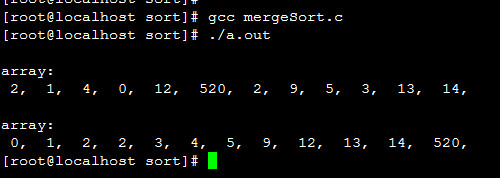时间复杂度

归并的时间复杂度分析：主要是考虑两个函数的时间花销，一、数组划分函数mergeSort()；二、有序数组归并函数_mergeSort()；

_mergeSort()函数的时间复杂度为O(n)，因为代码中有2个长度为n的循环（非嵌套），所以时间复杂度则为O(n)；

简单的分析下元素长度为n的归并排序所消耗的时间 T[n]：调用mergeSort()函数划分两部分，那每一小部分排序好所花时间则为  T[n/2]，而最后把这两部分有序的数组合并成一个有序的数组_mergeSort()函数所花的时间为
O(n)；

公式：T[n]  =  2T[n/2] + O(n)；

公式就不仔细推导了，可以参考下： 排序算法之快速排序及其时间复杂度和空间复杂度里面时间复杂度的推导；

所以得出的结果为：T[n] = O( nlogn )

因为不管元素在什么情况下都要做这些步骤，所以花销的时间是不变的，所以该算法的最优时间复杂度和最差时间复杂度及平均时间复杂度都是一样的为：O(
nlogn )；好像有人说最差的时间复杂度不是O(nlogn)，我不知道怎么算出来的，知道的麻烦告知下，谢谢；

空间复杂度

归并的空间复杂度就是那个临时的数组和递归时压入栈的数据占用的空间：n + logn；所以空间复杂度为: O(n)

以时间换空间

我看到网上很多blog分享空间复杂度只有O(1)的归并排序法；因为传统的归并排序所消耗的空间主要是在归并函数（把两个有序的函数合并成一个有序的函数），所以如果要让时间复杂度为 O(1)  ，那么也只能在归并函数中做文章了。代码就不列出来了，其主要思想就是借助于快速排序（其实就是相当于归并函数被快速排序函数替换了）；这样的方法虽然可以减少内存的消耗，但是却会在时间上带来损失，因为这样时间复杂度却变成了
O(n^2)  了；所以这种方法并不是一个两全其美的idea；

总结

归并排序虽然比较稳定，在时间上也是非常有效的（最差时间复杂度和最优时间复杂度都为 O(nlogn)  )，但是这种算法很消耗空间，一般来说在内部排序不会用这种方法，而是用快速排序；外部排序才会考虑到使用这种方法；

转载请注明作者和原文出处，原文地址：http://blog.csdn.net/yuzhihui_no1/article/details/44223225

若有不正确之处，望大家指正，共同学习！谢谢！！！

展开全文• 排序算法之 快速排序时间复杂度分析 排序算法之 堆排序及时间复杂度分析 归并排序 归并排序是建立在归并操作上的一种有效排序算法，该算法是采用分治法的一个典型应用。 这里的分治如何理解？ 比如我们要统计本...

排序算法之 冒泡排序及性能优化（时间复杂度+空间复杂度分析）
排序算法之 简单选择排序及时间复杂度分析
排序算法之 直接插入排序及时间复杂度分析
排序算法之 希尔排序及时间复杂度分析
排序算法之 快速排序及时间复杂度分析
排序算法之 堆排序及时间复杂度分析

归并排序
归并排序是建立在归并操作上的一种有效排序算法，该算法是采用分治法的一个典型应用。
这里的分治如何理解？
比如我们要统计本县城的高考状元，而一个县城中有很多中学，每个中学又有若干个班级，每个班级有若干名学生，每个学生是一个单独个体，看成数组中的一个元素。接下来，班级内学生两两组合并排好序组成一组，然后两组两组再进行合并并排好序…各班级分别排好序…各学校分别排好序…县中所有学生排序…
归并排序基本思想：

将序列中待排序数分为若干组，每个数字为一组
将若干个组进行两两合并，保证合并后的组是有序的
一直重复第二步操作直到剩下一组，排序完成

效果图：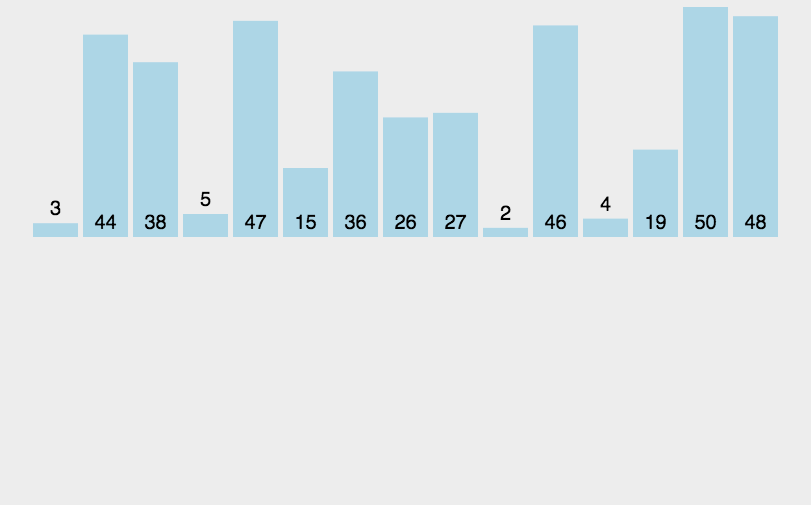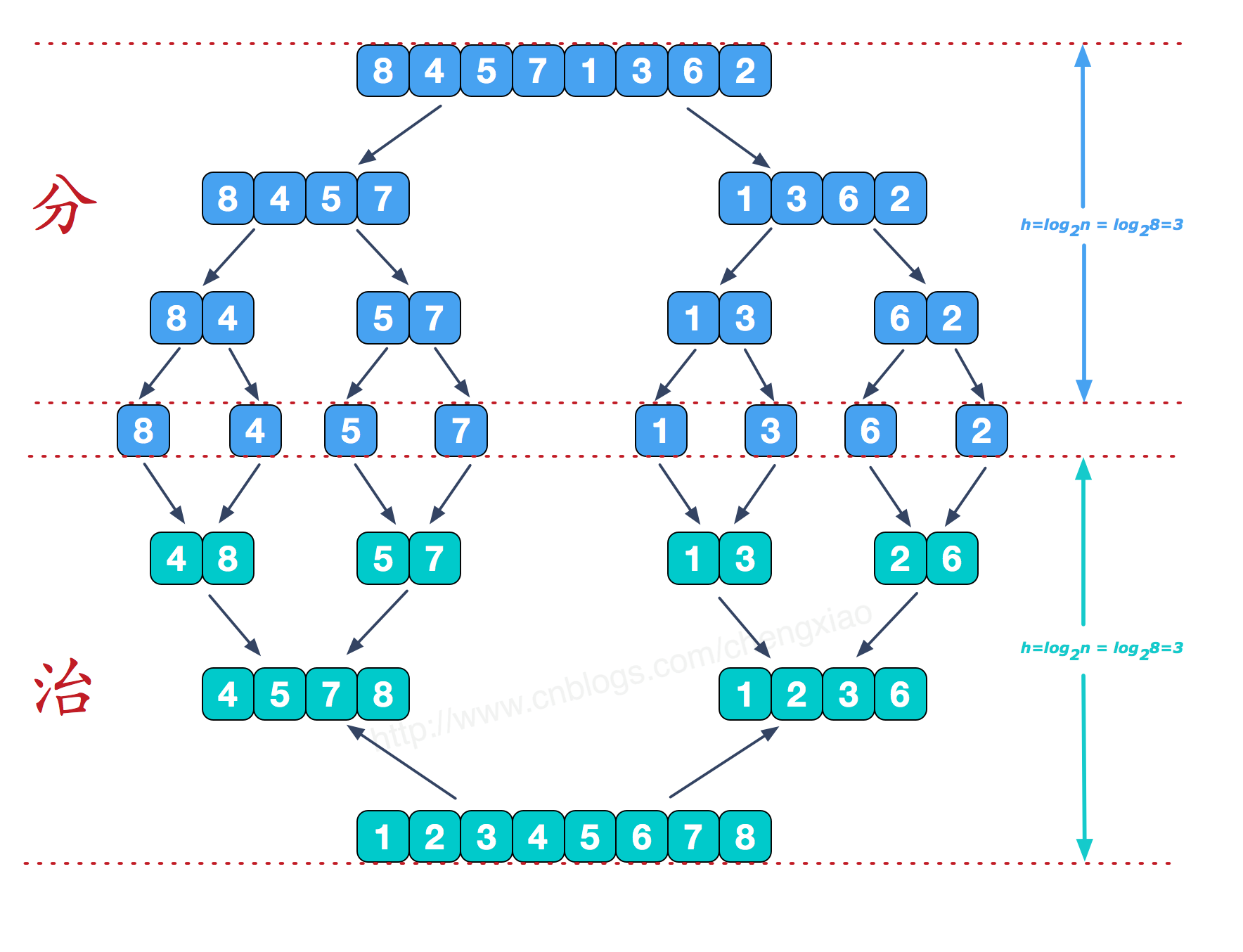算法实现
#include <stdio.h>

int arr1 = {9,8,7,6,5,4,3,2,1,0}, arr2;//原数组arr1，临时空间数组arr2
void merge(int low, int mid, int high) {
int i = low, j = mid + 1, k = low;
while (i <= mid && j <= high)
if (arr1[i]<arr1[j])
arr2[k++] = arr1[i++];
else
arr2[k++] = arr1[j++];
while (i <= mid)
arr2[k++] = arr1[i++];
while (j <= high)
arr2[k++] = arr1[j++];
for (i = low; i <= high; i++) {
arr1[i] = arr2[i];
}
}

void mergeSort(int a, int b) {
//直到a=b时，停止递归。
if (a<b) {
int mid = (a + b) / 2;
mergeSort(a, mid);
mergeSort(mid + 1, b);
merge(a, mid, b);
}
}

int main() {
int i;
mergeSort(0, 9);
return 0;
}


时间复杂度
归并排序的效率是比较高的，设数列长为N，将数列分开成小数列一共要logN步，每步都是一个合并有序数列的过程，时间复杂度可以记为O(N)，故一共为O(NlogN)。因为归并排序每次都是在相邻的数据中进行操作，所以归并排序在O(NlogN)的几种排序方法（快速排序，归并排序，希尔排序，堆排序）也是效率比较高的。


展开全文算法 数据结构
• 1. 归并排序快速排序 归并排序和快速排序的区别
1. 归并排序

归并排序是利用递归与分治技术将数据序列分成为越来越小的半子表，再对半子表排序，最后再用递归方法将排好序的半子表合并成为越来越大的有序序列。

归并排序算法的原理：

对于给定的一组记录（假设共有 n 个记录），首先将每两个相邻的长度为 1                             的子序列进行归并，得到 n/2 （向上取整）个长度为 2 或 1 的有序子序列，再将其两两归并，反复执行此过程，知道得到一个有序序列。

所以，归并排序的关键是两步：第一步，划分半子表；第二步，合并半子表。以数组 {49,38,65,97,76,13,27} 为例，归并排序的具体步骤如下：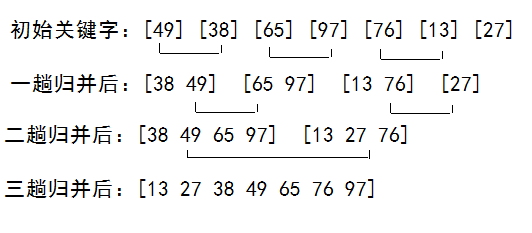程序示例如下：

public class SortMerge {

public static void Merge(int[] array, int p, int q, int r) {
int i,j,k,n1,n2;
n1 = q - p + 1;
n2 = r-q;
int[] L = new int[n1];
int[] R = new int[n2];
for(i = 0, k = p; i < n1; i++, k++)
L[i] = array[k];
for(i = 0, k = q + 1; i < n2; i++, k++)
R[i] = array[k];
for(k = p, i = 0, j = 0; i < n1 && j < n2; k++) {
if(L[i] < R[j]) {
array[k] = L[i];
i++;
}
else {
array[k] = R[j];
j++;
}
}
if(i < n1) {
for(j = i; j < n1; j++, k++)
array[k] = L[j];
}
if(j < n2) {
for(i = j; i < n2; i++, k++)
array[k] = R[i];
}
}
public static void MergeSort(int[] array, int p, int r) {
if(p < r) {
int q = (p + r)/2;
MergeSort(array, p, q);
MergeSort(array, q+1, r);
Merge(array, p, q, r);
}
}
public static void main(String[] args) {
int i = 0;
int a[] = {5,4,9,8,7,6,0,1,3,2};
int len = a.length;
MergeSort(a, 0, len-1);
for(i = 0; i < len; i++)
System.out.print(a[i] + " ");
}
}


二路归并排序的过程需要进行 logn 趟。每一趟就是讲两个有序子序列进行归并，而每一对有序子序列归并时，记录的比较次数等于记录的移动次数，记录移动的次数均等于文件中记录的个数 n，即每一趟归并的时间复杂度为 O(n)。因此，二路归并排序的时间复杂度为 O(nlogn)。

2. 快速排序

快速排序是一种非常高效的排序算法，它采用“分而治之”的思想，把大的拆分为小的，小的在拆分为更小的。

其原理如下： 对于一字给定的记录，通过一趟排序后，将原序列分为两部分，其中前一部分的所有记录均比后一部分的所有记录小，然后再一次对前后两部分的记录进行快速排序，递归该过程，指导序列中所有记录均有序为止。

具体而言，算法步骤如下：
1） 分解。将输入的序列 array[m … n] 划成两个非孔子序列 array[m … k] 和 array[k+1 … n]，使 array[m … k] 中任一元素的值不大于 array[k + 1 … n]中任一元素的值。

2）递归求解。通过递归调用快速排序算法分别对 array[m … k] 和 array[k+1 … n]进行排序。

3）合并。由于对分解出的两个子序列的排序是就地进行的，所以在 array[m … k] 和 array[k+1 … n] 都排好序后不需要执行任何计算 array[m … n]就已排好序。

以数组 {49,38,65,97,76,13,27,49}为例，整个排序过程如下所示：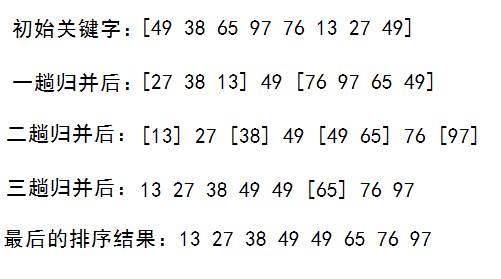程序示例如下：

public class SortQuick {
public static void sort(int[] array, int low, int high) {
int i, j;
int index;
if(low >= high)
return;
i = low;
j = high;
index = array[i];
while(i < j) {
while(i < j && array[j] >= index)
j--;
if(i < j)
array[i++] = array[j];
while(i < j && array[i] < index)
i++;
if(i < j)
array[j--] = array[i];
}
array[i] = index;
sort(array, low, i-1);
sort(array, i+1, high);
}
public static void quickSort(int[] array) {
sort(array,0,array.length-1);
}
public static void main(String[] args) {
int i = 0;
int[] a= {49,38,65,97,76,13,27,49};
quickSort(a);
for(i = 0; i < a.length; i++) {
System.out.print(a[i] + " ");
}
}
} /* Output:
13 27 38 49 49 65 76 97
*///~

当初始的序列整体或局部有序，快速排序的性能就会下降，此时，快速排序将退化为冒泡排序。

快速排序的相关特点如下：

1) 最坏时间复杂度。最坏情况是指每次区间划分的结果都是基准关键字的左边（右边）序列为空，而另一边区间中的记录项仅比排序前少了一项，即选择的基准关键字是待排序的所有记录中最小或最大的，例如，如果选取第一个记录为基准关键字，当初始序列按递增顺序排列时，每次选择的基准关键字都是所有记录中的最小者，这时记录与基准关键字的比较次数会增多。因此，在这种情况下，需要进行（n-1）次区间划分。对于第 k（0<k<n<!--//--><![CDATA[//><!--
0< k< n
//--><!]]>）次区间划分，划分前的序列长度为（n-k+1），需要进行（n-k）次记录的比较。因此，当 k 从 1 到 （n-1）时，进行的比较次数总共为 n(n-1)/2，所以，在最坏情况下快速排序的时间复杂度为 O（n^2）。

2) 最好时间复杂度。最好情况是指每次区间划分结果都是基准关键字左右两边的序列长度相等或相差为 1，即选择的基准关键字为待排序的记录中的中间值。此时，进行比较的次数总共为 nlogn，所以，在最好情况下快速排序的时间复杂度为 O（nlogn）。

3) 平均时间复杂度。快速排序的平均时间复杂度为 O（nlogn）。虽然快速排序在最坏情况下的时间复杂度为 O（n^2），但是在所有平均时间复杂度为 O（nlogn）的算法中，快速排序的平均性能是最好的。

4) 空间复杂度。快速排序的过程中需要一个栈空间来实现递归。当每次对区间的划分都比较均匀时（即最好情况），递归树的最大深度为 ⌈logn⌉+1<!--//--><![CDATA[//><!--
\left \lceil logn\right \rceil+1
//--><!]]>（logn为向上取整）；当每次区间划分都使得有一边的序列长度为 0 时（即最坏情况），递归树的最大深度为 n。在每轮排序结束后比较基准关键字左右的记录个数，对记录多的一边先进行排序，此时，栈的最大深度可降为 logn。因此，快速排序的平均空间复杂度为 O（logn）。

5) 基准关键字的选取。基准关键字的选取是决定快速排序算法性能的关键。常用基准关键字的选取方式如下：

（1）三者取中。三者取中是指在当前序列中，将其首、尾和中间位置上的记录进行比较，选择三者的中值作为基准关键字，在划分开始前交换序列中的第一个记录与基准关键字的位置。

（2）取随机数。取 left（左边）和 right（右边）之间的一个随机数 m（left⩽m⩽right<!--//--><![CDATA[//><!--
left \leqslant m \leqslant right
//--><!]]>），用 n[m] 作为基准关键字。这种方法使得 n[ left ] 和 n[ right ] 之间的记录是随机分布的，采用此方法得到的快速排序一般称为随机的快速排序。

3. 归并排序和快速排序的区别

快速排序和归并排序的原理都是基于“分而治之”思想，即首先把待排序的元素分成两组，然后分别对这两组排序，最后把两组结果合并起来。

它们的区别在于，进行的分组策略不同，后面的合并策略也不同。

归并排序的分组策略是假设待排序的元素存放在数组中，那么其把数组前面一半元素作为一组，后面一半作为另一组。 快速排序是根据元素的值来分组，即大于某个值的元素放在一组，而小于的放在另外一组，该值称为基准。所以，对整个排序过程而言，基准值的挑选非常重要，如果选择不合适，太大或太小，那么所有元素都分在一组了。

总的来说，快速和归并排序，如果分组策略越简单，后面的合并策略就越复杂，因为快速排序在分组时，已经根据元素大小来分组了，而合并时，只需要把两个分组合并起来就行了，归并排序则需要对两个有序的数组根据大小合并。
展开全文递归 合并
• 归并排序快速排序时间复杂度均为O(nlogn)，适合大规模的数据排序且很常用，它们都用到了分治思想，将大的问题分解成小问题来解决。接下来我们分别对其进行分析。 归并排序（Merge Sort） 思路：归并排序的核心...
• 排序算法分析2--归并排序和快速排序0.综述1.归并排序原理分析2.归并排序代码实现 0.综述 在前面一节中，我们分析了三种时间复杂度为O(n²)的排序算法–冒泡排序、插入排序、选择排序，由于这三种算法的时间复杂度较...
• 因为堆排序时间复杂度为n*logn，空间复杂度为1，是不...而归并排序时间复杂度为n*logn，空间复杂度为n，是稳定排序。 快速排序时间复杂度为n，空间复杂度最好的情况是logn，最坏的情况是n^2，是不稳定的排序方法
• 1、归并排序 归并排序算法的核心思想：把数组从中间分成前后两部分，然后对前后两部分分别排序，再将排好序的两部分合并在一起，这样整个数组就都有序了。具体过程如下图所示： 归并排序使用的就是分治思想。分治，...算法 数据结构 排序算法 java
• 时间复杂度 平均情况 时间复杂度 最好情况 时间复杂度 最坏情况 空间复杂度 插入排序 是 O(n²) O O(n²) O 希尔排序 （Shell） 是...
• 归并和快速排序都是利用分治的思想来把大问题拆分成一个个子问题，子问题都解决了，大问题也就解决了。 归并排序 归并排序算法的求解过程可以解释为层层递进式的将数列折半，当数列无法折半的时候（也就是数列内仅...排序算法
• 归并排序和快速排序总结1. 算法基本思路：分治分治，字面上的解释是“分而治之”，就是把一个复杂的问题分成多个同等结构的子问题，再把子问题分成更小的子问题……直到最后子问题可以简单的直接求解，原问题的解即...算法
• 本篇文章讲解三个高级排序算法，分别为希尔排序、归并排序快速排序。虽然它们的思想很复杂，但真的运用得非常得巧妙，我会用丰富的例子以及动图来让大家轻松地理解并掌握。算法 排序算法 javascript
• 算法的时间复杂度用来衡量随问题规模n的增长执行次数的增长率。 常见排序： 插入排序 遍历数组，遍历到i时，a0,a1...ai-1是已经排好序的，取出ai，从ai-1开始从后向前每个比较大小(从前向后比的话需要考...
• ## 归并排序和快速排序

千次阅读 多人点赞 2019-04-16 15:25:51
一，归并排序 归并排序算法实现 算法思路： 如果要排序一个数组，我们先从数组中间把数组分成左数组右数组两部分，分别对左右数组进行排序，然后将排序好的数组合并成结果数组，排序就完成了，最后只需将结果数组...排序 数据结构 算法
• 归并排序和快速排序，是两种时间复杂度为O(nlogn)的排序，适合大规模的排序，比上节所说的三种排序（冒泡、插入、选择）更常用 归并排放的原理 归并排序（Merge Sort ) 的核心思想还是蛮满意的。如果要排序一个...算法 递归
• （待会儿你会发现，即便是快速排序，最坏情况下，时间复杂度也是 O(n^2)。）但是，归并排序并没有像快排那样，应用广泛，这是为什么呢？因为它有一个致命的“弱点”，那就是归并排序不是原地排序算法。 这是因为归并...数据结构
• 归并排序和快速排序1.1 归并排序归并排序的思想就是讲数组分为两部分然后对两部分进行排序,然后讲排序后的两部分进行合并,主要的难度在于合并部分,合并的时候需要重新开一个临时数组保存合并的结果,然后再复制到原...python
• 归并排序快速排序都是基于比较的排序算法。本文将详细分析这两种排序。 先说结论 算法 基于比较 原地排序 稳定性 时间复杂度 最好 时间复杂度 最坏 时间复杂度 ...稳定性
• 如何计算归并排序时间复杂度？ 什么是归并排序归并排序的概念十分简单，就是“分而治之”的思想。这里我直接从网上找了一份对归并排序算法的比较好的介绍排序算法 。 计算时间复杂度 关键是怎么计算时间复杂度...排序算法 数据结构 算法
• 【算法复习2】时间复杂度 O[nlogn]快速排序归并排序分析归并排序稳定性递归转递推时间复杂度很稳定归并致命的空间复杂度快速排序快排 规则原地排序 超越归并缺点快排性能分析总结 归并排序 分治思想 分解 递归 对半...
• 各种排序算法的稳定性，时间复杂度和空间复杂度总结：我们比较时间复杂度函数的情况：时间复杂度函数O(n)的增长...2.线性对数阶O(nlog2n)排序快速排序、堆排序和归并排序；3.O(n1+§)排序，§是介于0和1之间的整数。...
• 归并排序快速排序都使用了分治的思想，时间复杂度都是O(N*logN)。由于其时间复杂度低，所以被广泛的应用，比如Java Collection.sort; Mysql数据库中的排序；Tim排序等。算法 排序算法
• 文章目录1. 基本思想2. 代码实现2.1 递归实现2.2 优化—非递归实现3...归并排序快速排序的思想基本一致,唯一不同的是归并排序的基准值是数组的中间元素 快排 Link：[排序算法] 6. 快速排序多种递归、非递归实现及性能
• 1.归并排序  也就是合并排序，将两个或两个以上的有序数据序列合并成一个新的有序数据序列，它的基本思想是假设数组A有N个元素，那么可以看成数组A有N个有序的子序列组成，每个子序列的长度为1，然后在将两两合并...
• 归并排序和快速排序都是采用递归的结构实现的，不同的是归并排序在递归过程中有合并子序列的过程，而快速排序中没有，但是快速排序中有较为复杂的划分过程。 二者的平均时间复杂度均为O(nlgn)，其中快速排序的系数...
• 快速排序 归并排序 基数排序 #include&amp;amp;amp;lt;stdio.h&amp;amp;amp;gt; #include&amp;amp;amp;lt;stdlib.h&amp;amp;amp;gt; #include&amp;amp;amp;lt;string.h&amp;amp;amp;gt...C C++...